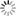# Episode List

OR

### Season 2

6 Jan. 1953
Island of StoneBe the first one to add a plot.

13 Jan. 1953
The ProsecutorBe the first one to add a plot.

20 Jan. 1953
The Beautiful Miss XBe the first one to add a plot.

27 Jan. 1953
Gambler's DebtBe the first one to add a plot.

3 Feb. 1953
I'll Never Know WhenBe the first one to add a plot.

10 Feb. 1953Be the first one to add a plot.

17 Feb. 1953
Second SonBe the first one to add a plot.

24 Feb. 1953
Mr. GeorgeBe the first one to add a plot.

3 Mar. 1953
Burden of GuiltBe the first one to add a plot.

10 Mar. 1953
A Letter for the QueenBe the first one to add a plot.

24 Mar. 1953
Night of FearBe the first one to add a plot.

31 Mar. 1953
Goodbye to the ClownBe the first one to add a plot.

7 Apr. 1953
The Dear DepartedBe the first one to add a plot.

21 Apr. 1953
Man Across the StreetBe the first one to add a plot.

28 Apr. 1953
No EscapeBe the first one to add a plot.

5 May 1953
The Worthless ThingBe the first one to add a plot.

12 May 1953
The Chinese StickBe the first one to add a plot.

18 May 1953
The Green and Gold StringBe the first one to add a plot.

26 May 1953
The Man on the BluffBe the first one to add a plot.

2 Jun. 1953Be the first one to add a plot.

9 Jun. 1953Be the first one to add a plot.

16 Jun. 1953
The Worried ManBe the first one to add a plot.

23 Jun. 1953
The Watchers and the WatchedBe the first one to add a plot.

30 Jun. 1953
Too Gloomy for Private PushkinItaly 1943, four US GI's, one Private (Pushkin) a Corporal and two Sergeants, volunteer to recce a heavily shelled castle. Owing to heavy fire, only two make it inside, the Corporal having turned back, while one Sergeant is killed at the door. Pushkin has a bad feeling about the castle being haunted, much to the amusement of the Sergeant, who remains intent of finding a 'bottle'! While checking out the cellar, Pushkin saves the life of the Sergeant in a fire fight with a German soldier, who had been looting treasures. Other German soldiers arrive and a further fire ...

7 Jul. 1953
Pattern in AlgiersBe the first one to add a plot.

14 Jul. 1953
Born That WayBe the first one to add a plot.

21 Jul. 1953
Death of a Smart AleckBe the first one to add a plot.

28 Jul. 1953
The Gold ThumbBe the first one to add a plot.

4 Aug. 1953Be the first one to add a plot.

11 Aug. 1953Be the first one to add a plot.

18 Aug. 1953
The Better HalfBe the first one to add a plot.

25 Aug. 1953
The Eye Witness Who Wouldn't SeeBe the first one to add a plot.

1 Sep. 1953
Last of the OutlawsBe the first one to add a plot.

8 Sep. 1953
Girl from KansasBe the first one to add a plot.

22 Sep. 1953
Incredible LuckBe the first one to add a plot.

29 Sep. 1953
Summer NightBe the first one to add a plot.

6 Oct. 1953
Night in the MuseumBe the first one to add a plot.

13 Oct. 1953
FaithBe the first one to add a plot.

20 Oct. 1953
Black PearlBe the first one to add a plot.

27 Oct. 1953Be the first one to add a plot.

3 Nov. 1953
The WesternerBe the first one to add a plot.

10 Nov. 1953
Guitar in GuatemalaBe the first one to add a plot.

17 Nov. 1953
Pretty PollyBe the first one to add a plot.

24 Nov. 1953
Lost KidBe the first one to add a plot.

1 Dec. 1953
The FBI StoryBe the first one to add a plot.

15 Dec. 1953
The Real Danger